# Principles of Optical Lenses

Apr. 22, 2022

Lenses are the optical components that make up the basic building blocks of many common optical devices, including cameras, binoculars, microscopes and telescopes. Lenses are essentially light control elements and are therefore used for light gathering and imaging. Curved mirrors and lenses can accomplish many of the same things in terms of light collection and image formation. However, lenses tend to be superior for imaging because they are transparent, which allows light to be transmitted directly along the axis to the detector, whereas mirrors require off-axis geometry. Mirrors are often preferred for light gathering because they can be manufactured to be much lighter than lenses, thus allowing for larger diameters and light gathering capabilities. This section discusses the refraction mechanisms that form the basis of lens operation, the issues that affect their performance, and the different lens types.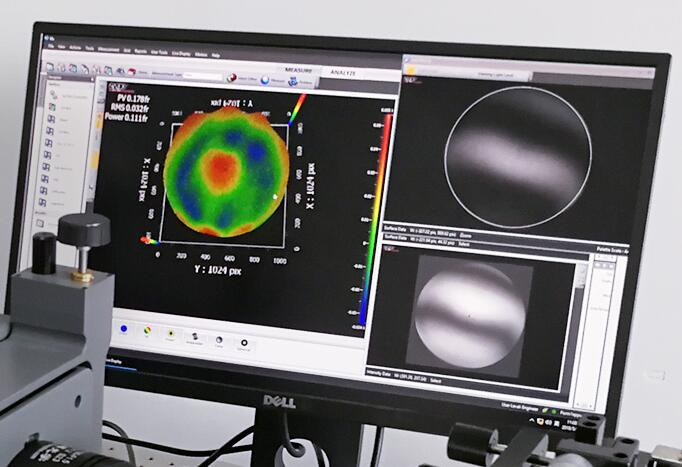## Refraction

In addition to light reflected from a flat interface between two media, it can be transmitted and then refracted in a second medium (see Figure 1). Refraction is the change in angle of the incident light as it enters the second medium. Since the speed of light in a medium is inversely proportional to its refractive index, it slows down or speeds up as it enters a different medium, causing the light to change direction. Figure 1 shows an example where the refractive index of the second medium (n2 ) is greater than that of the first medium ( n1 ), which causes the light to bend in the direction normal to the interface. This refraction phenomenon is described by Snell's law.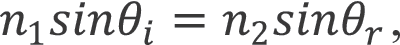where θ i and θ r are the angle of incidence and the angle of refraction, respectively (see Figure 94). If the direction of the incident light and the refractive index are known, Snell's law can determine the direction of refraction. This basic formula determines how the lens controls the light transmission for collection and imaging purposes.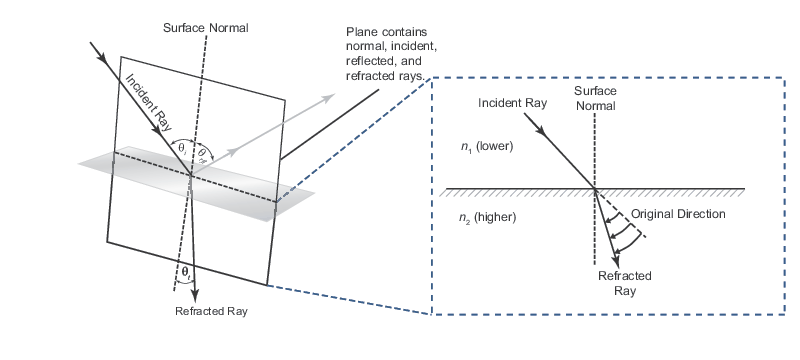Figure 1. shows Snell's law of refraction at the interface between a medium with refractive indices n 1 and n 2.

Lenses are typically made of transparent dielectric materials, such as fused silica or optical glass, with a spherical curvature on the front and back surfaces. Since the surface is curved, each ray of light parallel to the optical axis (as shown in Figure 2) has a different value of θ i relative to the surface normal. Each ray is then refracted according to Snell's law. For a positive lens, this causes the rays to converge to the focal point located on the right side of the lens, while the rays will diverge from the focal point located on the left side of the negative lens. The consequence of these operations is that the lens can be used for image formation as well as light collection and collimation (see Figure 2). There are several important aspects of using a lens for optical imaging, including the relationship between object distance and image distance, as well as the resulting magnification and the quality of the resulting image.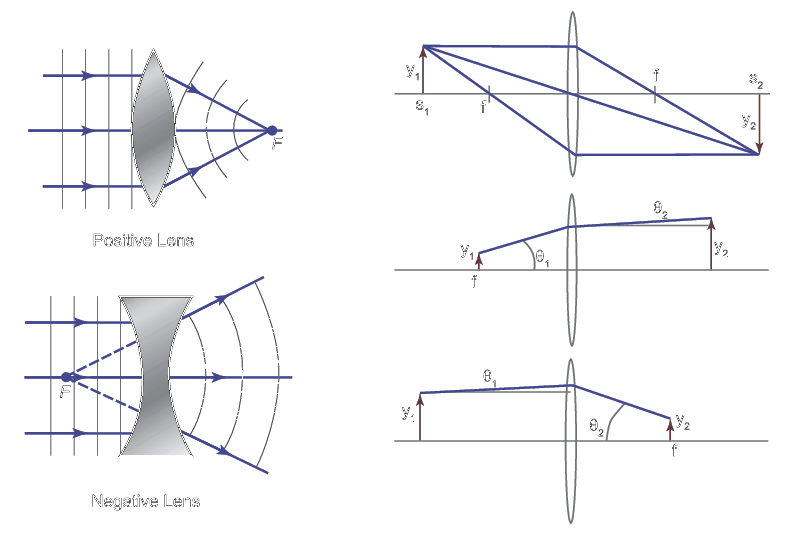Figure 2 illustrates how a lens affects the incident parallel light (left). Applications of the lens (right) include creating a magnified image of the object (top), collimating light from a point source (middle), and focusing a collimated light source (bottom).

## Optical aberration and lens type

The ideal lens will form a perfect image (or an exact replica of the object being imaged) and be able to focus collimated light to a spot size limited only by diffraction. However, true lenses are not perfect and can cause optical aberrations that result in a reduced ability to form high quality images, collimated beams, or tight focus. Monochromatic aberrations, which are wavelength-independent, are common for both mirrors and lenses and arise from the inability of the sphere to focus light properly when it is far from the axis. These aberrations include spherical aberration, coma, and image dispersion. Figure 3 illustrates the effect of spherical aberration in a lens, where light from small angles is effectively collimated, while light from large angles converges instead. Unlike monochromatic aberration, chromatic aberration occurs only in the lens. Due to the dispersion of the refractive index of the lens material, different wavelengths are refracted at different angles according to Sneer's law (see Figure 3). When using broadband light, this can lead to a reduction in image quality or focusing power.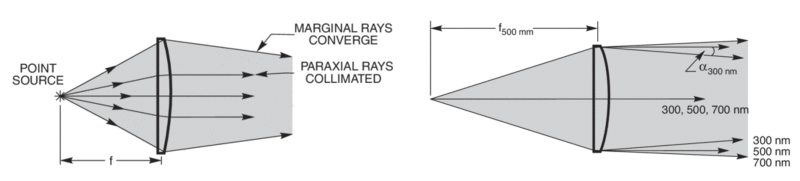Figure 3. The effect of spherical (left) and chromatic aberration (right) on collimation when the point source is in focus.

While spherical lenses do produce aberrations, choosing the right lens shape can help minimize optical aberrations (see Figure 4). For example, a plano-convex lens that is curved on only one side is the best choice for focusing parallel light rays to a single point. A biconvex lens (where the curvature on both sides may not be equal) is the best choice for imaging when the object and image are at similar distances from the lens. Aspheric lenses may be overused when a single spherical lens may not be suitable due to spherical aberration. These lenses have custom curvature on the surface to help minimize the effects of aberration, but are typically expensive due to the complexity associated with manufacturing. Alternatively, multiple spherical lenses can be used, where one lens can eliminate the aberration caused by another lens, as shown in Figure 4. In addition to correcting for monochromatic aberrations, achromatic doublet lenses can be used to minimize chromatic aberrations by selecting the dispersion of the material to produce wavelength-independent focal lengths in both lenses. Microscope objectives are multi-element lens systems that can significantly reduce the effect of aberration but are more expensive due to the complexity of the design. All of the above lenses are rotationally symmetric, meaning that the light is focused identically regardless of which horizontal axis it crosses. Cylindrical lenses, on the other hand, focus or extend light on only one axis, making them ideal for modifying an asymmetric beam (such as the output of a laser diode) into a symmetric beam. Many characteristics need to be considered when selecting a lens, including focal length, lens shape, F/#, lens material, transmission characteristics, wavefront aberration, scattered light, coating type, and cost.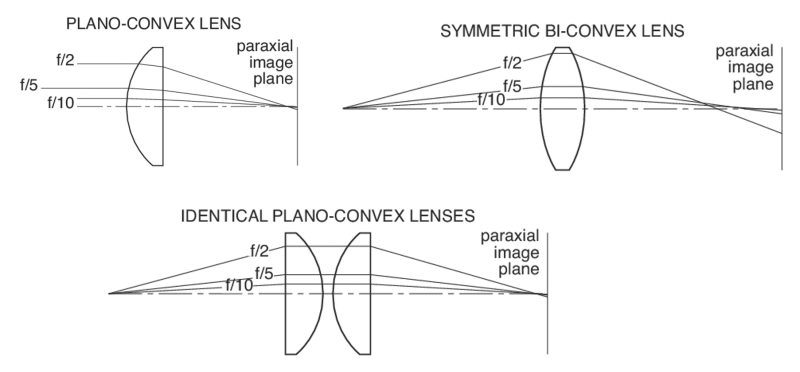Figure 4. Minimizing optical aberrations for specific imaging applications using single and multi-lens systems.